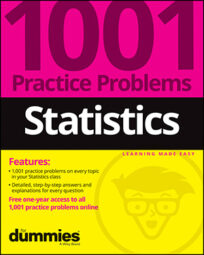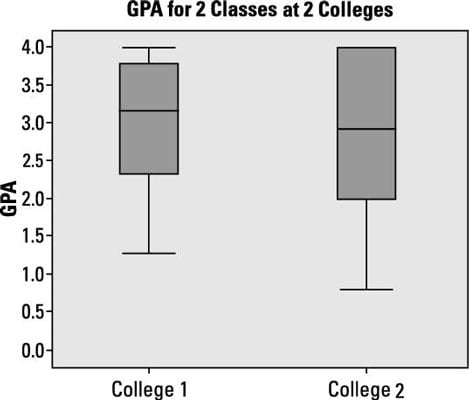##### Statistics: 1001 Practice Problems For Dummies (+ Free Online Practice)When working on statistics problems, you probably will have occasion to compare two box plots. The following box plots represent GPAs of students from two different colleges, call them College 1 and College 2.Credit: Illustration by Ryan Sneed

## Sample questions

1. What information is missing on this graph and on the box plots?

(A) the total sample size

(B) the number of students in each college

(C) the mean of each data set

(D) Choices (A) and (B)

(E) Choices (A), (B), and (C)

Answer: E. Choices (A), (B), and (C) (the total sample size; the number of students in each college; the mean of each data set)

The sample size isn’t accessible from a box plot. You know that 25% of the data lies within each section, but you don’t know the total sample size. You also don’t know the mean; you see the median (the line inside the box), but the mean isn’t included on a box plot.

2. Which data set has a greater median, College 1 or College 2?

The median is indicated by the line within the actual box part of the box plot. Comparing the medians, you can see College 1’s median has a greater value than College 2’s.

3. Which data set has the greater IQR, College 1 or College 2?

The interquartile range (IQR) is the distance between the 3rd and 1st quartiles and represents the length of the box. If you compare the IQR of the two box plots, the IQR for College 2 is larger than the IQR for College 1.

4. Which data set has a larger sample size?

Answer: Impossible to tell without further information.

Just because one box plot has a longer box than another one doesn’t mean it has more data in it. It just means that the data inside the box (the middle 50% of the data) is more spread out for that group. Each section marked off on a box plot represents 25% of the data; but you don’t know how many values are in each section without knowing the total sample size.

5. Which data set has a higher percentage of GPAs above its median?

Answer: The two data sets have the same percentage of GPAs above their medians.

The median is the place in the data set that divides the data in half: 50% above and 50% below. So both data sets have 50% of their GPAs above their respective medians.

If you need more practice on this and other topics from your statistics course, visit 1,001 Statistics Practice Problems For Dummies to purchase online access to 1,001 statistics practice problems! We can help you track your performance, see where you need to study, and create customized problem sets to master your stats skills.